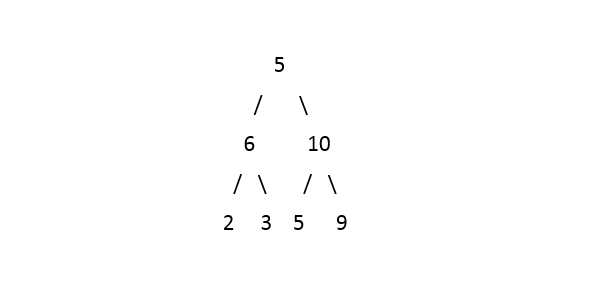5

# Odd even level

Difficulty: EASY
Avg. time to solve
20 min
Success Rate
87%

Problem Statement
Suggest Edit

#### Given a binary tree. Find and return the modulus of the difference between the sum of odd level nodes and the sum of even level nodes.

##### Input format:
``````The first line contains an integer 'T' which denotes the number of test cases or queries to be run. Then, the test cases follow.

The first and only line of every test case contains elements in the level order form. The line consists of values of nodes separated by a single space. In case a node is null, we take -1 on its place.

For example, the input for the tree depicted in the below image would be :
````````````1
2 3
4 -1 5 6
-1 7 -1 -1 -1 -1
-1 -1
``````

#### Explanation :

``````Level 1 :
The root node of the tree is 1

Level 2 :
Left child of 1 = 2
Right child of 1 = 3

Level 3 :
Left child of 2 = 4
Right child of 2 = null (-1)
Left child of 3 = 5
Right child of 3 = 6

Level 4 :
Left child of 4 = null (-1)
Right child of 4 = 7
Left child of 5 = null (-1)
Right child of 5 = null (-1)
Left child of 6 = null (-1)
Right child of 6 = null (-1)

Level 5 :
Left child of 7 = null (-1)
Right child of 7 = null (-1)

The first not-null node (of the previous level) is treated as the parent of the first two nodes of the current level. The second not-null node (of the previous level) is treated as the parent node for the next two nodes of the current level and so on.
The input ends when all nodes at the last level are null (-1).
``````
##### Note :
``````The above format was just to provide clarity on how the input is formed for a given tree.
The sequence will be put together in a single line separated by a single space. Hence, for the above-depicted tree, the input will be given as:

1 2 3 4 -1 5 6 -1 7 -1 -1 -1 -1 -1 -1
``````
##### Output format:
``````For each test case, print an integer denoting the modulus of the difference in a single line.
``````
##### Constraints:
``````1 <= T <= 5
0 <= N <= 10^5
0 <= val <= 10^9

Time limit: 1 sec
``````
##### Sample Input 1:
``````5 6 10 2 3 5 9 -1 -1 -1 -1 -1 -1 -1 -1
``````
##### Sample Output 1:
``````8
``````

Explanation``````Level 1= {5}, Sum= 5
Level 2= {6, 10} Sum= 16
Level 3= {2, 3, 5, 9}, Sum=19
Odd sum = Sum of Level 1 + Sum of Level 3
5 + 19
24

Even sum = Sum of Level 2
16

| Even sum - Odd sum | = 8
``````
##### Sample Input 2:
``````5 3 9 2 6 5 10 -1 -1 -1 -1 -1 -1 -1 -1
``````
##### Sample Output 2:
``````16
``````